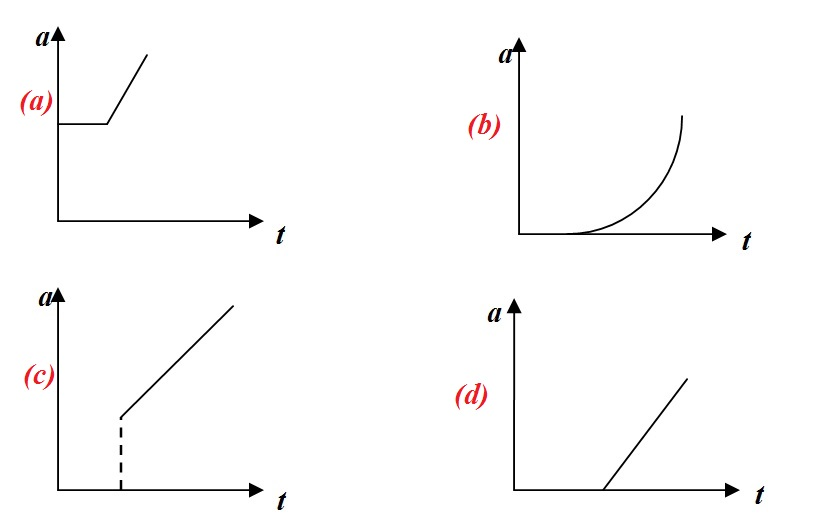# Graphs-1(Physics)A block is placed on a rough horizontal plane. A time-dependent horizontal force $F=kt$ acts the block, where $t$ is time and $k$ is a positive constant. Which of the following represents the acceleration-time graph of the block?

Details

• The block has a coefficient of static friction $\mu_s$ with the surface, and a smaller coefficient of kinetic friction $\mu_k$. Also Try my Easy Mechanics Set Easy Mechanics
×